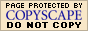Completing the Square:
Deriving the Vertex Formula
(page 2 of 2)

Since you always do exactly the same procedure each time you find the vertex form, the procedure can be done symbolically (using y = ax2 + bx + c instead of putting in numbers), so you end up with a formula that you can use instead of doing the completing-the-square process each time. Here's what it looks like:

• Derive the formula for the x-coordinate "h" of the vertex of any quadratic.

 This is your generic quadratic equation. y = ax2 + bx + c Move the loose number over to the other side. y – c = ax2 + bx Factor out whatever is multiplied on the squared term.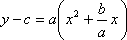Make room on the left-hand side, and put a copy of "a" in front of this space. Take half of the coefficient of the x-term (divide it by two) (and don't forget its sign!); square this, and add it inside the parentheses on both sides.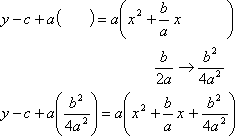Multiply through the parentheses on the left.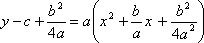Simplify on the left (converting to common denominators, if necessary), and convert to squared form on the right.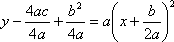Move the loose numbers back over to the right-hand side.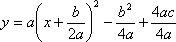Convert to vertex form, as necessary.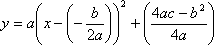Then the the vertex (h, k) for any given quadratic y = ax2 + bx + c obeys the formula: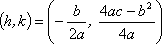Practically speaking, you can just memorize that h = b (2a) and then plug your value for "h" back in to "y =" to calculate "k". If you're allowed to use this formula, you can then more quickly find the vertex, because simply calculating h = b (2a) and then finding k is a lot faster than completing the square. On the other hand, even if you're not "supposed" to use this formula, you can still use it to check your work. If you're not sure if you completed the square correctly on a test, you can always use the formulaic answer to verify (or correct) whatever you've come up with.

However, if you've memorized the Quadratic Formula, you can probably easily memorize the formula here for k. The discriminant in the Quadratic Formula is b2 – 4ac. Take the negative of this to get the reverse of the subtraction:

–(b2 – 4ac) = 4acb2

The formula for h has a denominator of 2a; double this for a denominator of 4a. Put them together, and this gives you the formula for k.